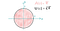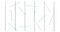# Estimating π with Monte-Carlo-Simulations

## The easy, the sophisticated and the surprising way of estimating π

Pi Day is coming up soon! And there are many ways to calculate or estimate our all-time favorite number π which is approximately 3.14159….

Let’s have a look at these methods and let’s discuss three ways to estimate π using Monte-Carlo Simulations!

# What is Pi?

It is the area of the unit circle (i.e. the circle with radius 1) or half the perimeter of the unit circle.A unit circle with area A(1) = π and perimeter U(1) = 2π.

Generally, the area of a circle is given by

and it’s perimeter by

So for a circle with radius 1, this means that the area is π and the perimeter is 2π.

This famous number π can be calculated in many ways. There are lots of deterministic methods (i.e. methods where no randomness is used), but there are also many probabilistic methods (a.k.a. lazy methods) to estimate this number π.

Such methods are called Monte-Carlo Simulations. Let’s first look at what a Monte-Carlo Simulation is before we dig into the math.

# What is a Monte-Carlo Simulation?

Monte-Carlo Simulations are experiments or computational algorithms that rely on sampling of random numbers. An experiment or a simulation of random numbers is repeated a large number of times to estimate something that may be determined deterministically as well (such as π, as it is a deterministic number, i.e. it does not depend on randomness or chance).

Monte-Carlo Simulations are used whenever calculating something in a deterministic fashion is too computationally expensive or not feasible anymore. Or when you’re just too lazy to compute something exactly.

And sometimes it is not even necessary to use Monte-Carlo Simulations and the only reason to use them is to show how beautiful Math and Probabilities can be.

So let’s use some randomness to estimate our favorite number π.

I’ll give you three versions to do this: The easy, the sophisticated and the surprising way.

Enjoy!

# 1. The Easy Way: The Unit Square and the Unit Circle

Draw a Unit Square just like here and randomly (uniformly distributed) draw n dots on it. Count the number of dots m that are within a distance of 1 from the origin, i.e. within the circle as shown here.

The ratio m/n will be the approximate ratio of area Aₘ to area Aₙ which is equal to 1/4 π.

Why? Let’s find out.

The area Aₙ of the little square in the first quadrant is given by

The area Aₘ of one-fourth of the unit circle is given by

Thus we can calculate π using the ratio of these two areas Aₘ and Aₙ

and estimate π by four times the ratio of Aₘ to Aₙ. for example if we draw 100 dots and 76 of them end up within the quarter circle, π is approximated by

Not too bad, is it? If we for example now draw 1000 dots and 780 of them lie within the quarter circle, this approximates π by

which is even better. We can make this more accurate by drawing more and more dots because thanks to the law of large numbers, we will eventually come very close to π. Thus, we have found our very first method to estimate π using randomness!

This is probably the easiest — and most obvious — way to estimate π. It’s also a nice way to understand Monte-Carlo-Simulations and great for teaching children at a young age as it does not involve a lot of math.

Let’s now have a look at another less obvious and mathematically more challenging way.

# 2. The Sophisticated Way: Averages of Functions

The average of a continuous function f(x) is given by

We can use this to estimate π because for the function

, the integral

equals π/4 and thus also the integral

equals π/4. Thus also the average value of this function on the interval [0,1] equals π/4.

Let’s calculate this mathematically. The antiderivative for f(x) is given by

It’s tedious work to double-check this, so it’s up to you to believe me or do the work. I’ll skip it here, but trust me, it’s true (Sorry!). We can now calculate the definitive integral, i.e. the average for the above function, by

Thus, to estimate π, we can sample n random numbers xᵢ, i=1,…n between 0 and 1, calculate f(xᵢ) for them and take the average. If we multiply this by four, it will approximately equal π.

Again, it’s quite a simple method to estimate π, even though the mathematical explanation behind it is a little bit more complex than in the previous version.

This is a commonly used method to estimate integrals, especially when the exact calculation of the integral is too expensive or finding the antiderivative is not possible.

Let’s now have a look at the promised third version to estimate π.

# 3. The Surprising Way: Throwing Sticks

Take a sheet of paper and draw parallel lines that have a distance of 1 inch (or whichever unit you prefer). Now take n needles (or sticks) also of length 1 inch (or your chosen unit). Throw these sticks onto the sheet of paper and count the number m of sticks crossing any of the lines.11 needles (or sticks) are thrown onto a sheet of paper with parallel lines on it. Of these 11 needles, 7 hit the lines, these are drawn in the turquoise color.

The ratio m/n is approximately the same as 2/π, i.e.

and thus we can estimate π by

In the above case, we have for example a total of 11 needles of which 7 cross a line. Thus π can be estimated by 2 ⋅ 11/7 ≈ 3.1428 which is quite a good approximation.

Surprisingly accurate, isn’t it? (Even though I have to admit, I drew the picture and only realized how lucky I was with the numbers afterward!).

Let’s understand why this works.

To understand this, let x be the center of a needle. Whether or not the needle hits a line will only depend on the orientation of the needle.

The needle may be oriented anywhere between 0 and 180 °, i.e. π radians. It will cross the line if it is within 2⋅ α(x) of that center.

For a radius r=1, the needle of length 1 will hit a line with a chance P that depends on the angle α(x). Half a circle has a radiant of π. The needle hits the line if we are within the pinkish area. The pinkish area takes a fraction of 2α(x)/π of the pink and turquoise area together and thus the chance of hitting the line is given by

Because for

the above integral can be calculated by

The easiest way to double-check this is to calculate the derivative of

But again, as this is tedious work, I won’t do the full math here and leave it to you to double-check this.

This method is especially beautiful because it is surprisingly simple to execute. It works very well, even though it may be a little bit harder to understand why it works compared to the other versions.

But when using this method to estimate pi, you could be sitting on a beach with nothing more than a piece of wood. And you would still get a surprisingly good estimate of π. And there’s beauty in that, isn’t there?

# Some Final Thoughts

It’s up to you to pick your favorite. It may be one of these three that I showed you here or a totally different one.

What’s your favorite method to estimate (or calculate) our all-time favorite number π?

Written by

## More From Medium

Apr 2 · 25 min read

### The Invention Of Zero

Apr 1 · 7 min read